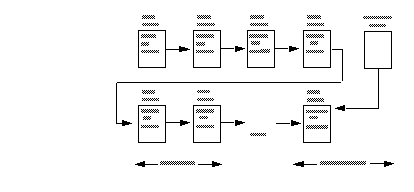# Create a Virtually Hashed Table

To create a virtually hashed table, specify the maximum value for the hash region.

This is the partial syntax for create table; the parameters for virtually hashed tables are shown in bold:
```create table [database.[owner].]table_name
. . .
| {unique | primary key}
using clustered
(column_name [asc | desc] [{, column_name [asc | desc]}...])=
(hash_factor [{, hash_factor}...])
with max num_hash_values key```
where:
• using clustered – indicates you are creating a virtually hashed table. The list of columns are treated as key columns for this table.

• column_name [asc | desc] – because rows are placed based on their hash function, you cannot use [asc | desc] for the hash region. If you provide an order for the key columns of virtually hashed tables, it is used only in the overflow clustered region.

• hash_factor – required for the hash function for virtually hashed tables. For the hash function, a hash factor is required for every key column. These factors are used with key values to generate hash value for a particular row.

• with max num_hash_values key – the maximum number of hash values that you can use. Defines the upper bound on the output of this hash function.

## Determining Values for hash_factor

You can keep the hash factor for the first key as 1. The hash factor for all the remaining key columns is greater than the maximum value of the previous key allowed in the hash region multiplied by its hash factor.

SAP ASE allows tables with hash factors greater than 1 for the first key column to have fewer rows on a page. For example, if a table has a hash factor of 5 for the first key column, after every row in a page, space for the next four rows is kept empty. To support this, SAP ASE requires five times the amount of table space.

If the value of a key column is greater than or equal to the hash factor of the next key column, the current row is inserted in the overflow clustered region to avoid collisions in the hash region.

For example, t is a virtually hashed table with key columns id and age, and corresponding hash factors of (10,1). Because the hash value for rows (5, 5) and (2, 35) is 55, this may result in a hash collision.

However, because the value 35 is greater than or equal to 10 (the hash factor for the next key column, id), SAP ASE stores the second row in the overflow clustered region, avoiding collisions in the hash region.

In another example, if u is a virtually hashed table with a primary index and hash factors of (id1, id2, id3) = (125, 25, 5) and a max hash_value of 200:
• Row (1,1,1) has a hash value of 155 and is stored in the hash region.

• Row (2,0,0) has a hash value 250 and is stored in overflow clustered region.

• Row (0,0,6) has a hash factor of 6 x 5, which is greater than or equal to 25, so it is stored in the overflow clustered region.

• Row (0,7,0) has a hash factor of 7 x 25, which is greater than or equal to 125, so it is stored in the overflow clustered region

This example illustrates how the number of rows in the hash region, row length, and the number of rows per page affect the page layout of hash and overflow regions. It creates a virtually hashed table named orders on the pubs2 database on the order_seg segment:
```create table orders(
id int,
age int,
primary key using clustered (id,age) = (10,1) with max 1000 key)
on order_seg```
The layout for the data is:
• The order_seg segment starts on page ID 51200.

• The logical page size is 2048 bytes

• The ID for the first data object allocation map (OAM) page is 51201.

• For the logical page size of 2048 bytes, the maximum rows per page is 168.

• The row size is 10.

• The root index page of the overflow clustered region is 51217.

In this example:
• The row size is 10 bytes

• 1000 rows fit in the hash region, with key values ranging from (0,0) to (99,9)

• The total number of pages in the hash region is 6, with 168 rows per page in the hash region and a maximum of 1000 keys (ceiling(1000/168) = 6). The last page (the sixth) has some unused space. Assuming the segment starts at page 51200 and the first extent is reserved for the OAM page, the first data page starts from 51208, so pages in the hash region range from 51208 to 51213.

The page after the last page in hash region (page number 51214) is the first page of the overflow region and is governed by a clustered index, so the root page, 51217, points to page number 51214.For this page layout, the number of rows per page is 168. Since the hash factors for id and age are 10 and 1, respectively, the maximum value for column age that qualifies for the hash region is 9. The range of key values of (id and age) combination that qualify for the the hash region (1000 keys in total) is:
• (0, 0) – (0, 9) – for a total of 10

• (1, 0) – (1,9) – for a total of 10

• (2, 0) – (2, 9) – for a total of 10

• . . .

• (99, 0) – (99, 9) – for a total of 10

From these keys, the first 168 keys—(0, 0) to (16, 7)—are mapped to the first data page, 51208. The next range of 168 keys—(16, 8) to (33, 5)—are mapped to the second data page, 51209, and so on.